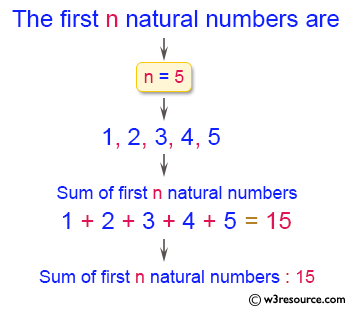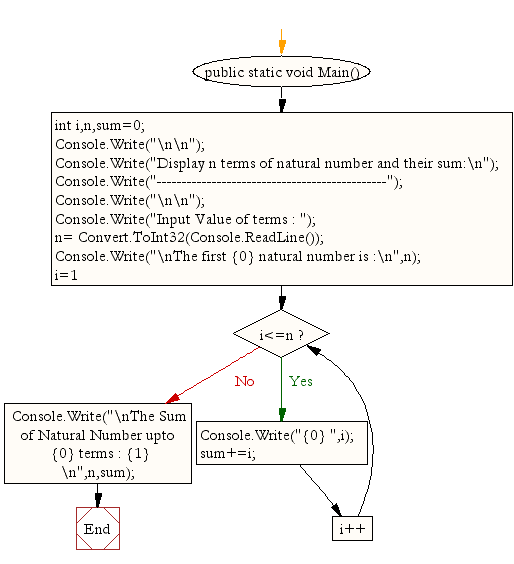﻿ C# - Display n natural numbers and their sum - w3resource# C# Sharp Exercises: Display n natural numbers and their sum

## C# Sharp For Loop: Exercise-3 with Solution

Write a program in C# Sharp to display n terms of natural number and their sum.

Pictorial Presentation:Sample Solution:

C# Sharp Code:

``````using System;
public class Exercise3
{
public static void Main()
{
int i,n,sum=0;

Console.Write("\n\n");
Console.Write("Display n terms of natural number and their sum:\n");
Console.Write("----------------------------------------------");
Console.Write("\n\n");

Console.Write("Input Value of terms : ");
Console.Write("\nThe first {0} natural number are :\n",n);
for(i=1;i<=n;i++)
{
Console.Write("{0} ",i);
sum+=i;
}
Console.Write("\nThe Sum of Natural Number upto {0} terms : {1} \n",n,sum);
}
}
```
```

Sample Output:

```Display n terms of natural number and their sum:
----------------------------------------------

Input Value of terms : 5

The first 5 natural number are :
1 2 3 4 5
The Sum of Natural Number upto 5 terms : 15
```

Flowchart:C# Sharp Code Editor:

Contribute your code and comments through Disqus.

What is the difficulty level of this exercise?

Test your Programming skills with w3resource's quiz.

﻿